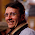## Wednesday, May 11, 2011

### Equilibrium index

The equilibrium index of an array is an index j such that the sum of the elements before j is equal to the sum of elements after j. Find j.

1.What about the below C++ code? Does that count as a solution? It returns -1, if no such index exists (I have not tested it, though :))

====

int equilibrium(const int *a, const int n)
{
int sumRight = 0;
for (int i = 0; i < n; ++i) sumRight += a[i];

int j, sumLeft = 0;
for (j = 0; j < n; ++j) {
if (sumLeft == sumRight) break;
sumLeft += a[j];
sumRight -= a[j];
}

return (j == n-1)?-1:j;
}

2.are you assuming it is sorted?